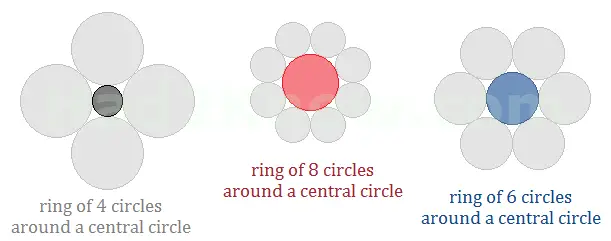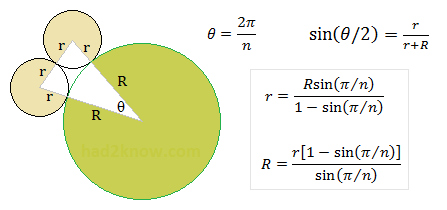# How to Find the Radius of a Circle Within a Ring of n Circles

 Outer Circular Ring Calculator Number of circles in outer ring = radius of outer circles = radius of center circle =

A popular type of geometric design is a symmetric ring of n equally sized circles arranged around central circle that is tangent to the ring. In order for the circles to touch properly without overlaps or gaps, you must know the exact radius of each circle.

The radius of the center circle depends upon the number n as well as the radius of each circle in the outer ring. Likewise, the radius of an outer ring circle depends on n and radius of the inner circle.

The formulas for calculating the radii are described below and require only a hand calculator to evaluate. You can also use the convenient calculator on the left. Simply enter the number of circles in the outer ring (n) and the radius of either the central or exterior circle(s). The calculator will compute the unknown dimensions of the other circle(s).

See images below for details on how the formulas are derived.To begin, call the radius of the inner circle R and the radius of the outer circles r. When n = 3, 4, or 5, the outer circles are larger. When n = 6, the inner and outer circles have equal radii. For all n larger than 6, the outside circles are smaller.

If you connect the centers of two adjacent exterior circles and the center of the central circle, you form an isosceles triangle with sides 2r, r + R, and r + R. The angle θ in the triangle has a measure of 2π/n radians (not degrees). The figure below shows the relations among r, R, and θ.If you know the value of R and n, you can compute r with the equation

r = Rsin(π/n)/[1 - sin(π/n)].

If you know the value of r and n, you can compute R with the equation

R = r[1 - sin(π/n)]/sin(π/n).

Example: Suppose you want to place 10 equal circles around a central circle that is 1 foot in diameter. Since n = 10 and R = 0.5 feet, you can compute the radius of the exterior circles with the expression

r = 0.5sin(π/10)/[1 - sin(π/10)]
= 0.5(0.309017)/(1 - 0.309017)
= 0.5(0.447214)
= 0.223607 feet, or 2.6833 inches.